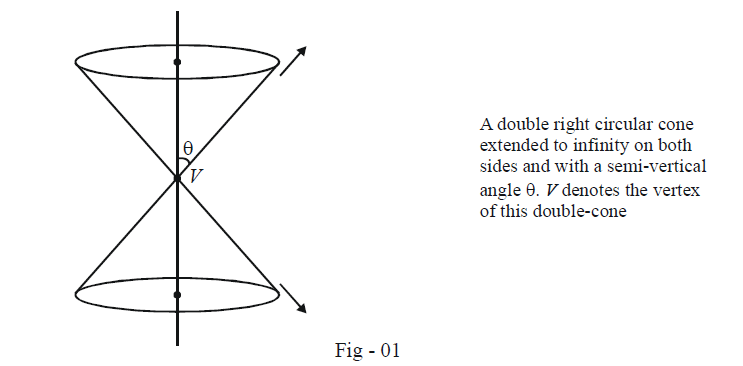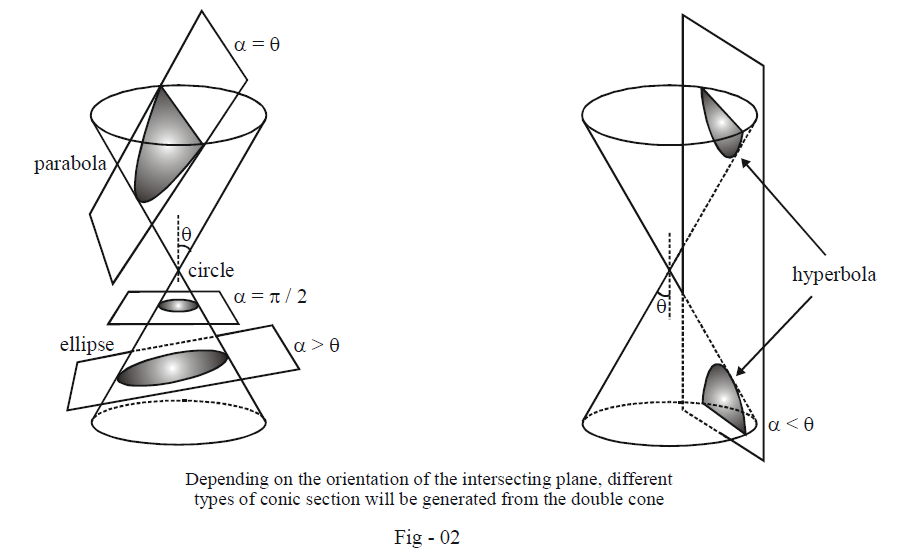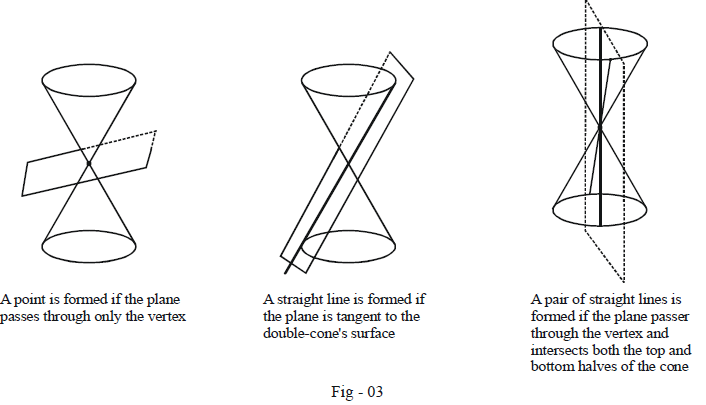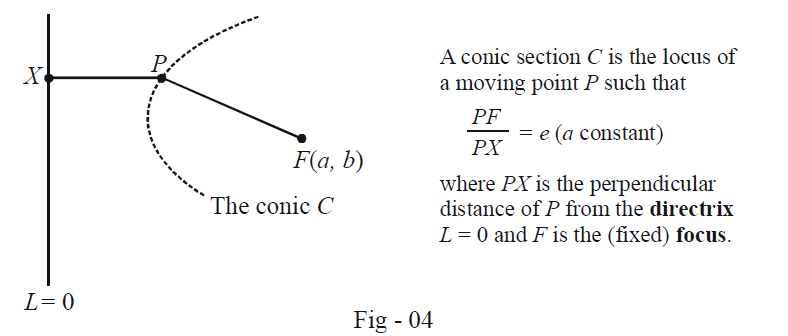# Introduction To Parabolas

Go back to  'Parabola'

Learn from the best math teachers and top your exams

• Live one on one classroom and doubt clearing
• Practice worksheets in and after class for conceptual clarity
• Personalized curriculum to keep up with school

# Parabola

In the course of the next three chapters, we’ll be studying conic sections, which as the name suggests, have something to do with sections of a cone. Different ways of cutting a cone using a plane will give rise to different conics.

We consider a (three-dimensional) double right circular as shown in the figure below, extended to infinity on both sides, and with a semi-vertical angle $$\theta$$.Now we use a plane to cut this double cone. Refer to the next figure and see that, depending on how we orient our plane, we give rise to

(a)  circles         -            we’ve already discussed circles in the previous chapter; we thus see that circles are a particular sort of conic sections with the intersecting plane at inclination $$\pi /2$$ .

(b)  parabola       -         we’ll discuss parabolas in this chapter; the intersecting plane for a parabola is parallel to the slant of the cone, i.e., at an angle $$\theta$$ .

(c)  ellipse           -            the intersecting plane is at an angle $$\alpha > \theta \,\,(\alpha \ne \pi /2$$ since then a circle will be formed)

(d)  hyperbola     -      the intersecting plane is at an angle $$\alpha < \theta ;$$ in this case, the plane cuts both the top and bottom halves of the coneThe next figure shows that even straight lines, pairs of straight lines and in fact even a point can be considered as sections of a cone. This is because these can be formed if the intersecting plane passes through the vertex of the double cone.These last three cases are termed degenerate cases of the conic sections. In our subsequent discussion, by conic sections we will always imply only the no-degenerate cases, i.e, circles, parabolas, ellipses or hyperbolas.

A very important property that a conic section C satisfies is this. It is the locus of a moving point P such that P's distance from a fixed point is always in a constant ratio to its (perpendicular) distance from a fixed line. The fixed point is called the focus of C while the fixed line is called the directrix of C. The constant ratio is called the eccentricity of C and is denoted by e.For a circle, the eccentricity e is 0 because while the fixed point (the focus) is the centre of the circle, the fixed line is assumed to be at infinity. Thus, PX in the figure above always remains infinitely large so that e = 0.

For the remaining conic sections, we have

 Parabola : e = 1 Ellipse : e < 1 Hyperbola : e > 1

Our discussions in this and the next two chapter deal separately with these three types of conics.

Parabolas
grade 11 | Questions Set 1
Parabolas
Parabolas
grade 11 | Questions Set 2
Parabolas